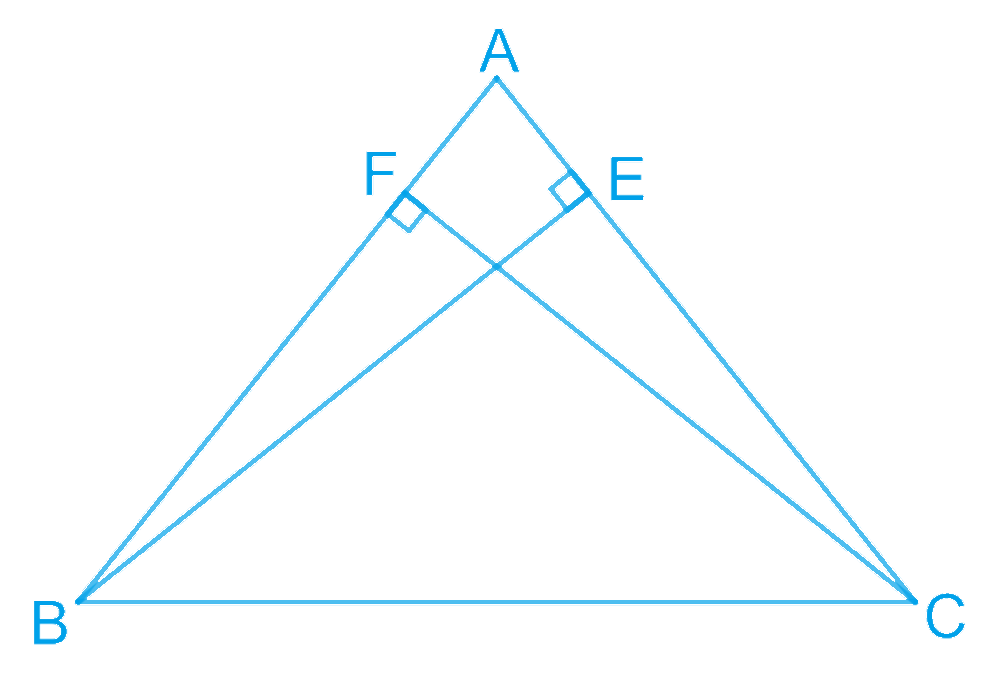# Ex.7.2 Q4 Triangles Solution - NCERT Maths Class 9

Go back to  'Ex.7.2'

## Question

$$ABC$$ is a triangle in which altitudes $$BE$$ and $$CF$$ to sides $$AC$$ and $$AB$$ are equal (see the given figure).

Show that:

(i) $$\ \Delta ABE \cong \Delta ACF$$

(ii) $$AB=AC,$$ i.e., ABC is an isosceles triangle.

Video Solution
Triangles
Ex 7.2 | Question 4

## Text Solution

What is Known?

Altitudes,$$\text{BE}=\text{CF},\text{ BE}\bot \text{AC}\ \text{and CF}\bot \text{AB}$$

To prove:

(i) $$\ \Delta ABE \cong \Delta ACF$$
(ii) $$AB=AC,$$ i.e., ABC is an isosceles triangle.

Reasoning:

We can show two triangles $$ABE$$ and $$ACF$$ congruent by using AAS congruency rule and then we can say corresponding parts of congruent triangles will be equal.Steps:

(i) In $$\Delta ABE\text{ and }\Delta ACF,$$

\begin{align}& \angle AEB \text{ and }\angle AFC \;(\text{Each} 90^{\circ}) \\ \angle A&=\angle A \;(\text{Common angle}) \\ BE&=CF \; (\text{Given}) \\ \therefore \Delta ABE &\cong \Delta ACF \\&(\text{By AAS congruence rule}) \end{align}

(ii) It has already been proved that

\begin{align}&\Delta ABE \cong \Delta ACF, \\ &\therefore AB = AC \; (\text{By }CPCT) \\ \end{align}

Video Solution
Triangles
Ex 7.2 | Question 4

Learn from the best math teachers and top your exams

• Live one on one classroom and doubt clearing
• Practice worksheets in and after class for conceptual clarity
• Personalized curriculum to keep up with school